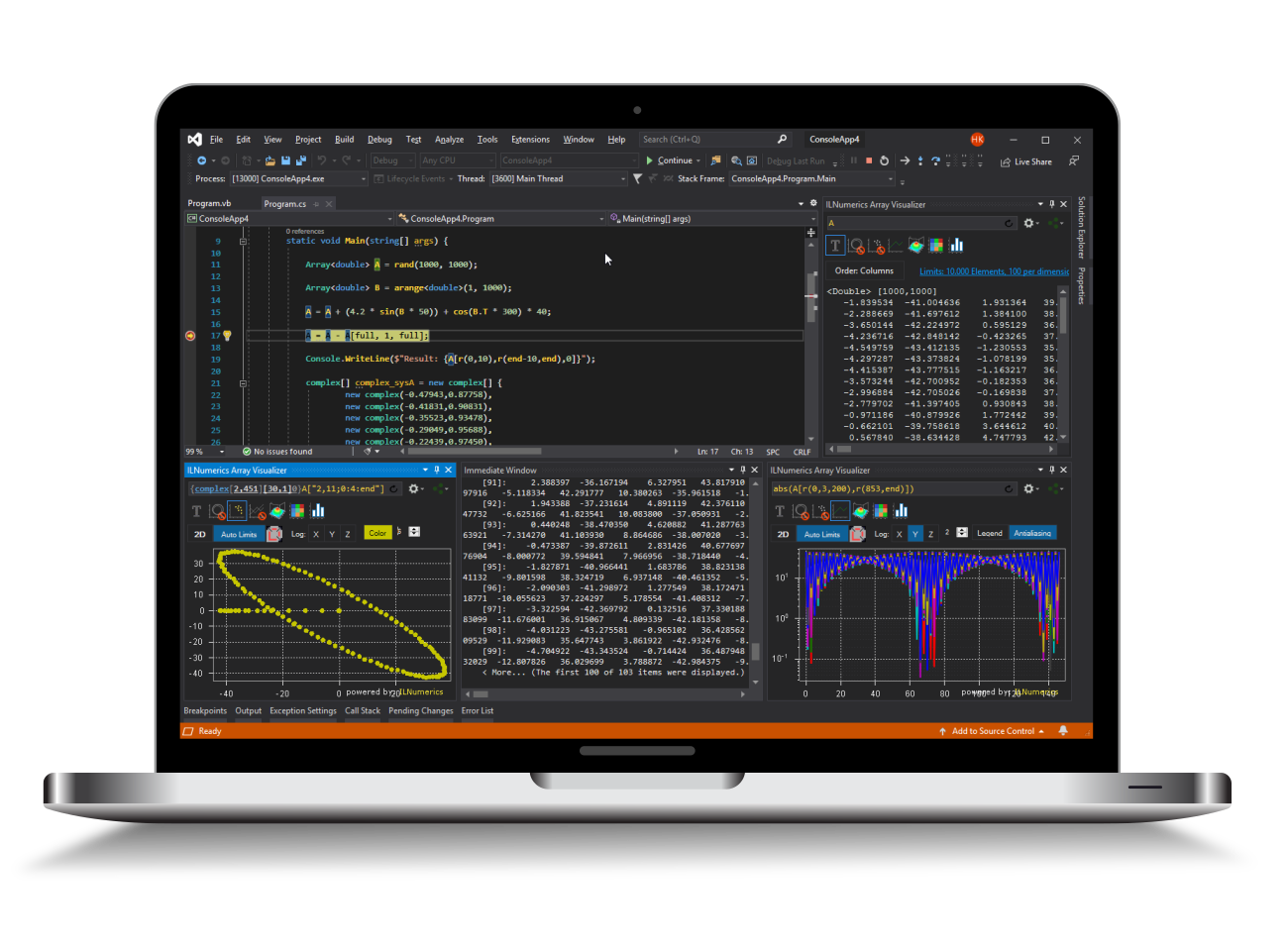Industrial Data Science
in C# and .NET:
Simple. Fast. Reliable.ILNumerics - Technical Computing

Modern High Performance Tools for Technical

Computing and Visualization in Industry and Science

tgt

# Binary Array Operators

Arrays can be combined by using common mathematical operators: +, -, *, /, and all comparison operators are defined. An expression C = A + B; adds corresponding elements of A and B and stores the result in C. The element type of all arrays involved must match. For the sizes of both arrays, the following combinations are allowed:

• Both arrays have the same size.
• Corresponding dimensions of both arrays must have either the same length or at least one of the dimensions must be a singleton dimension (i.e.: length equals 1). Read on for details.

The general broadcasting replaces the vector expansion feature as by ILNumerics version 4.10.

The general broadcasting implementation allows to apply a binary operation on two arrays even in the case that the shapes and number of elements of both arrays do not match. However, the shapes must be suitable to transform one or both arrays into a shape which does match. 'Suitable' here means: if the lengths of an unmatching dimension differ for two arrays, it must be scalar (or singleton, i.e.: length = 1) for at least one of both arrays.

Such transformation has the same effect to an array as replicating the array along the singleton dimension in order to match the length of the other dimension.

Example: Broadcasting a vector to make it suitable for a binary operation with a matrix.

This scheme is easily generalized to the n-dimensional case. Following examples of array combinations are all suitable for broadcasting. Note that the general suitability of scalar arrays is a direct consequence of the general broadcasting rules. Note further, that for ILNumerics arrays unspecified trailing dimensions are considered singleton dimensions.

The general broadcasting in ILNumerics is implemented in a very efficient way. Internally, in general no memory is replicated for most cases. While the broadcasting gives the same effect as if repmat would have been applied, the result is computed in a very different way and no new memory is used up for it.

By default and for compatibility reasons ILNumerics does not broadcast two vector sized arrays. Providing a row vector and a column vector to a binary function does not expand the singleton dimensions of both vectors to a matrix. Instead the operation is done on the existing vector elements only, the result, again, is a vector. Both input arguments are required to have the same length. An exception is thrown otherwise.

A new configuration variable has been introduced in version 4.10: Settings.BroadcastCompatibilityMode [default: true]. By default this switch is set to true, disabling the broadcasting for two vector arguments of different shape, providing legacy bahavior. One can set this switch to false in order to enable broadcasting for the special situation of two vector arguments also:

## Operators and static Functions

The following table lists all operators defined for ILNumerics arrays. Static functions are provided for languages not supporting overloaded operators.

Operation Operator static function ILMath.[...] Allowed Element Types
Subtraction - subtract(A,B) all numeric
Negation - (unary minus) invert(A,RetVal) all signed numeric
Multiplication         (element wise) * multiplyElem(A,B) all numeric
Matrix Multiplication [N/A] multiply(A,B) float,double, (f)complex
Division / divide(A,B) all numeric
Comparison Operators
Lower than < lt(A,B) all numeric
Lower equal <= le(A,B) all numeric
Greater than > gt(A,B) all numeric
Greater equal >= ge(A,B) all numeric
Equals == eq(A,B) all numeric
Not equal to != neq(A,B) all numeric

Bitwise Operators
Bitwise Left Shift << lshift(A,B)

Int32, UInt32, Int64

Bitwise Right Shift >> rshift(A,B) Int32, UInt32, Int64
Bitwise Or [N/A] bitor(A,B) Int32, UInt32, Int64
Bitwise And [N/A] bitand(A,B) Int32, UInt32, Int64
Bitwise Xor [N/A] bitxor(A,B)

Int32, UInt32, Int64

The element type for both operands must be the same for binary operators. Conversion functions exist (convert(), todouble(), tosingle(), toint32() a.s.o) which are useful for converting element types.

The element type of the return value will always be the same as the element type of the operands or a logical array in case of comparison operations.

### Limitations for Binary Bitwise Operators

Binary bitwise shifting operators << and >> are one exception to the above rule. Here, the C# language specification requires the second operand to be of type Int32. Therefore, the operators << and >> are defined for Array<T> with integer T and a scalar second operator B only. The corresponding lshift() and rshift() functions can be used directly if you need elementwise shifting of two non-scalar operands.

The C# operators | (bitwise or) and & (bitwise and) are not applicable to Array<T>. Overriding them would conflict with the || operator which is used for combining Logical arrays and considered more important. Use the corresponding functions bitor() and bitand() instead. The same is true for the ^ (xor) operator. Here, bitxor() is to be used.

## Custom Binary Operations

The apply() function comes in handy if you want to chain / customize arbitrary elementwise operations on two arrays. Here, some examples are given:

## Logical Binary Functions

All logical comparison operators return logical arrays. Refer to the section on logical arrays for details.